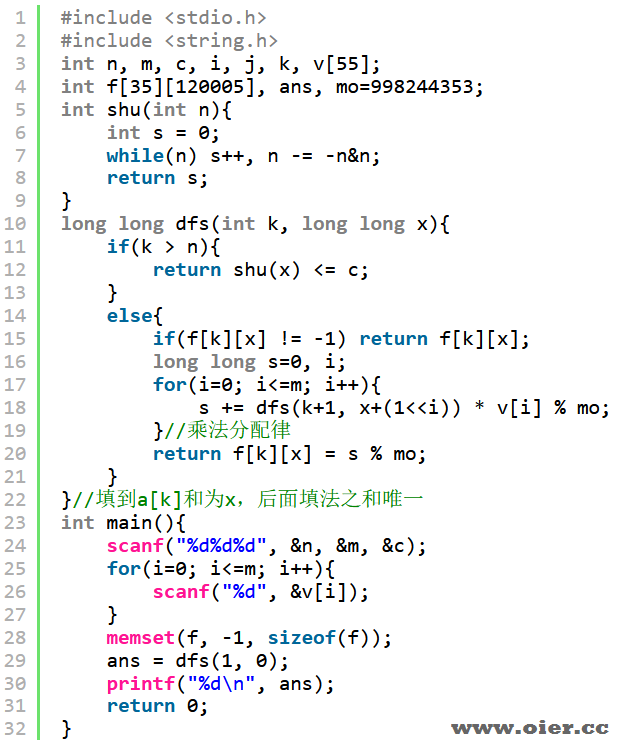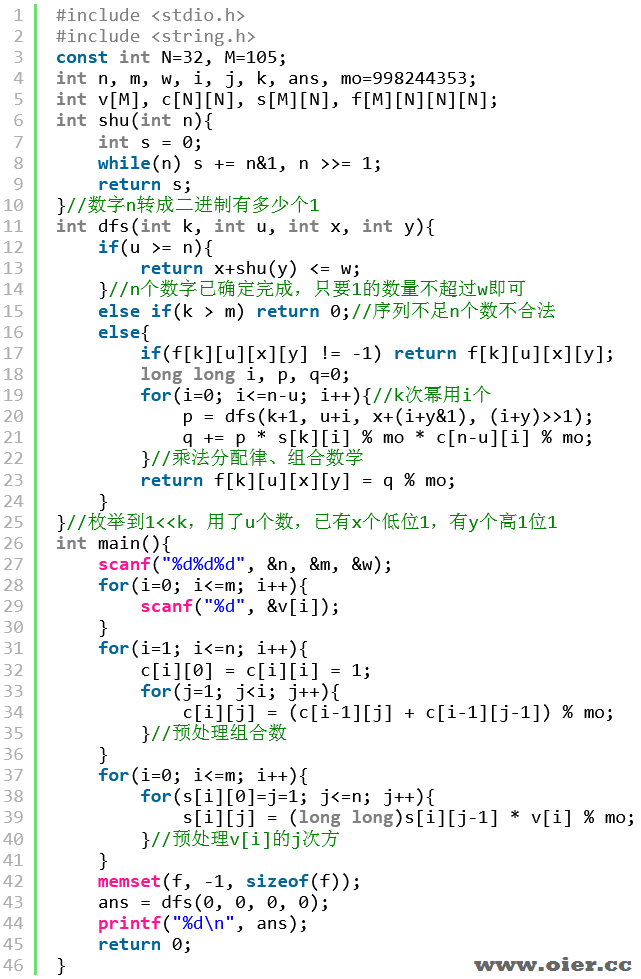1245+

## 输入输出样例

### 输入样例 #1

5 1 1
2 1


### 输出样例 #1

40


### 输入样例 #2

见附件中的 sequence/sequence2.in

### 输出样例 #2

见附件中的 sequence/sequence2.ans

## 说明

**【样例解释 #1】**

**【数据范围】**

| 测试点 | $n$ | $k$ | $m$ |
| :———-: | :—: | :——: | :—-: |
| $1 \sim 4$ | $=8$ | $\leq n$ | $=9$ |
| $5 \sim 7$ | $=30$ | $\leq n$ | $=7$ |
| $8 \sim 10$ | $=30$ | $\leq n$ | $=12$ |
| $11 \sim 13$ | $=30$ | $=1$ | $=100$ |
| $14 \sim 15$ | $=5$ | $\leq n$ | $=50$ |
| $16$ | $=15$ | $\leq n$ | $=100$ |
| $17 \sim 18$ | $=30$ | $\leq n$ | $=30$ |
| $19 \sim 20$ | $=30$ | $\leq n$ | $=100$ |

## 程序实现（感谢mekoszc提供考场代码）